## Why Generic?

``````fun acceptString(obj: String): String {
println("acceptString:\$obj")
return obj
}

fun acceptInt(obj: Int): Int {
println("acceptString:\$obj")
return obj
}
``````

``````val str = acceptString("100")
println("acceptString output:" + str.toInt().plus(10)) // acceptString output:110

val int = acceptInt(100)
println("acceptInt output:" + int.plus(10)) // acceptInt output:110
``````

### 試試看 Any

``````fun acceptAny(obj: Any): Any {
println("acceptAny:\$obj")
return obj
}
``````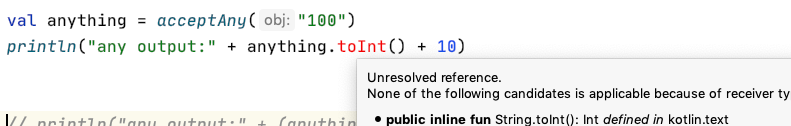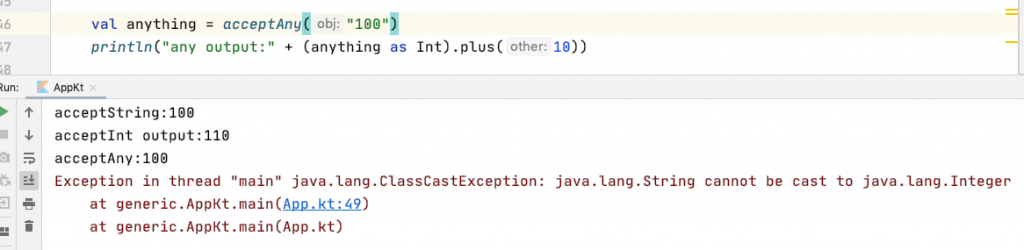``````val anything = acceptAny("100")
println("any output:" + (anything as String).toInt().plus(10)) // any output:110
``````

## Generic (泛型) 會是你的救星

``````fun <T> acceptT(obj: T): T {
println("acceptT:\$obj")
return obj
}
``````
``````// call generic
val t = acceptT("100")
println("generic output:" + t.toInt().plus(10)) // generic output:110
``````

## Generic function (泛型函數)

`<T>` 就是我們要使用的泛型的型態代號，不一定要使用Ｔ，要用什麼英文字母都可以，按照以往的慣例會用大寫字母表示，一般來說會用Ｔ來宣告。

### 兩種泛型型態，輸入和回傳的型態不一樣

``````fun <T, R> acceptTAndReturnR(obj: T): R {
return "test" as R
}
``````

### mutableMapOf() 的原始碼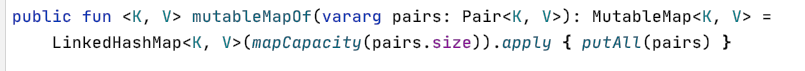## Generic class (泛型類別)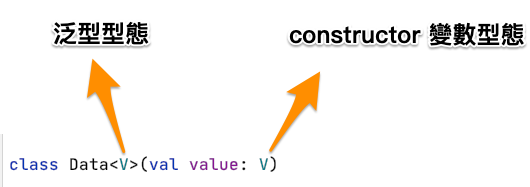``````val dataLong = Data<Long>(1000L)
val dataStr = Data<String>("data test")
``````

## Type parameter constraints (泛型型態的限制）

### 如果想要設定泛型型態的`上界(upper bound)`

``````fun <T: Number> sum(num1: T, num2: T) :T {
return num1.toDouble().plus(num2.toDouble()) as T
}
``````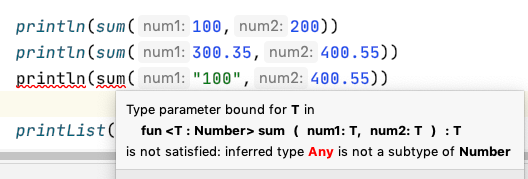### 如果想要多個`上界(upper bound)`

`fun <T> appendDot(seq: T): T` 這部分是正常泛型宣告

`where T : CharSequence, T : Appendable` 這部分就定義了兩個上界，限定 T 要是 `CharSequence``Appendable` 這兩個 interface 的物件實作，才能傳入這個函數

``````fun <T> appendDot(seq: T): T
where T : CharSequence, T : Appendable {
if (!seq.endsWith('.')) {
seq.append('.')
}
return seq
}
``````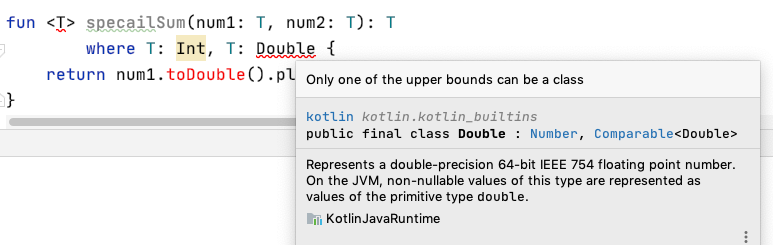## 隱藏的上界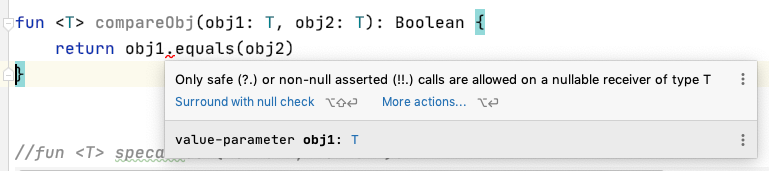``````fun <T> compareObj(obj1: T, obj2: T): Boolean? {
return obj1?.equals(obj2)
}
``````

``````fun <T : Any> compareObj2(obj1: T, obj2: T): Boolean? {
return obj1.equals(obj2)
}
``````